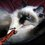My favorite number is: $3435$. You may ask: "why?" since this number seems, à priori, pretty boring. But it turns out that it is very interesting: it is the only natural number (along with $1$ ) which have the following property: \begin{aligned}\color{#3D99F6}3\color{#D61F06}4\color{#20A900}35&=\color{#3D99F6}3^{\color{#3D99F6}3}+\color{#D61F06}4^{\color{#D61F06}4}+\color{#20A900}3^{\color{#20A900}3}+5^5\\&= \color{#3D99F6}{27}+\color{#D61F06}{256}+\color{#20A900}{27}+3125\\ &={3435}.\end{aligned} I also like Mills' constant which is the smallest number $\rm A$ such that $\lfloor \rm A^{3^n} \rfloor$ is a prime number for every $n\in\mathbb N$. Its value is approximately equal to $1.306$ and the primes generated by Mills' constant are known as Mills primes; if the Riemann hypothesis is true, the sequence begins: $2, 11, 1361, 2521008887, \ldots$ (sequence A051254 in OEIS). And what about you? What is your favorite number, and why? Leave a comment! ;-)Note by حكيم الفيلسوف الضائع
6 years, 5 months ago

This discussion board is a place to discuss our Daily Challenges and the math and science related to those challenges. Explanations are more than just a solution — they should explain the steps and thinking strategies that you used to obtain the solution. Comments should further the discussion of math and science.

When posting on Brilliant:

• Use the emojis to react to an explanation, whether you're congratulating a job well done , or just really confused .
• Ask specific questions about the challenge or the steps in somebody's explanation. Well-posed questions can add a lot to the discussion, but posting "I don't understand!" doesn't help anyone.
• Try to contribute something new to the discussion, whether it is an extension, generalization or other idea related to the challenge.

MarkdownAppears as
*italics* or _italics_ italics
**bold** or __bold__ bold
- bulleted- list
• bulleted
• list
1. numbered2. list
1. numbered
2. list
Note: you must add a full line of space before and after lists for them to show up correctly
paragraph 1paragraph 2

paragraph 1

paragraph 2

[example link](https://brilliant.org)example link
> This is a quote
This is a quote
    # I indented these lines
# 4 spaces, and now they show
# up as a code block.

print "hello world"
# I indented these lines
# 4 spaces, and now they show
# up as a code block.

print "hello world"
MathAppears as
Remember to wrap math in $$ ... $$ or $ ... $ to ensure proper formatting.
2 \times 3 $2 \times 3$
2^{34} $2^{34}$
a_{i-1} $a_{i-1}$
\frac{2}{3} $\frac{2}{3}$
\sqrt{2} $\sqrt{2}$
\sum_{i=1}^3 $\sum_{i=1}^3$
\sin \theta $\sin \theta$
\boxed{123} $\boxed{123}$

Sort by:

4, because I used to think that numbers had personalities. 4 is kind.

- 6 years, 5 months ago

1. My family literally revolves around 5 and any number who's ultimate digit sum is 5.

- 6 years, 5 months ago

I was born on the fifth hour of the fifth day of the fifth month of the year on the fifth floor of the hospital, and I used to be 5 years old. That's why my favorite number is $145=1!+4!+5!$.

- 6 years, 5 months ago

$1023$ because...

• In alphanumeric code, it spells my initials
• $2^{10}-1$ looks nice
• Contains digits 0 through 3
• It was especially special on October 23, 2013
• 10:23 is my average bedtime

- 6 years, 5 months ago

8... because in a digital clock all the digits and also the letters can be formed by 8.. for example- to form 3 in a digital clock we just have to take out the two sticks on the left...

- 6 years, 5 months ago

73, from the Big Bang Theory

- 6 years, 5 months ago